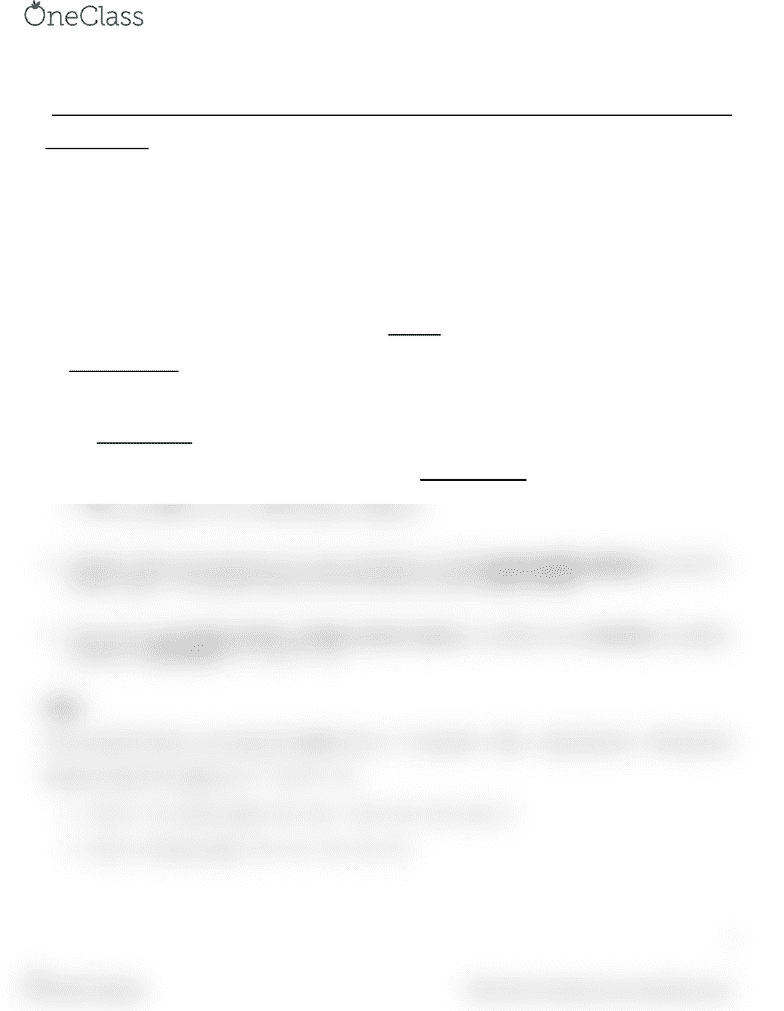# MGOC10H3 Lecture Notes - Lecture 2: Feasible Region, Sensitivity Analysis

70 views2 pages
School
Department
Course
Professor

For unlimited access to Class Notes, a Class+ subscription is required.1
MGOC10 Analysis for Decision Making
Lecture 02
Chapter 2 Solving LP Model Graphical Method & Sensitivity Analysis of Constraints
LP terminology
Example Giapetto’s problem
MAX Z = 3X1 + 2X2
S.T. 2X1 + X2 100 (finishing)
X1 + X2 80 (carpentry)
X1 40 (soldier demand)
X1 0 , X2 0
Any specification of values for variables is called a solution eg. X1 = 60, X2 = 10.
Feasible solution is a solution that satisfies all constraints and sign restrictions
eg. [X1 = 40, X2 = 20] or [X1 = 30.5, X2 = 20.001] or …
The feasible region is set of all feasible solutions.
Given many feasible solutions, goal is to find the best or optimal solution.
For max problem, optimal (best) solution is one which maximizes value of objective.
For min problem, it is one which minimizes objective.
Solutions which are intersection of 2 or more constraints are called corner feasible solutions (at corner of
feasible region). The optimal solution for an LP must be a corner feasible solution.
LPs can have one optimal solution, multiple optimal solutions, or problem can be infeasible (no feasible
solution) or unbounded ( Z = ∞ or Z = - ∞)
Slack
Given optimal solution, can compute the slack for any “≤” constraints. Slack = right hand side left hand side.
Optimal solution for Giapetto, X1* = 20, X2* = 60
For X1 + X2 80 hrs, Slack = 80 (X1* + X2*) = 80 (20 + 60) = 0.
For X1 40 units, Slack = 40 X1* = 40 20 = 20.
find more resources at oneclass.com
find more resources at oneclass.com
Unlock document

This preview shows half of the first page of the document.
Unlock all 2 pages and 3 million more documents.

Already have an account? Log in

# Get access

Grade+
\$10 USD/m
Billed \$120 USD annually
Homework Help
Class Notes
Textbook Notes
40 Verified Answers
Study Guides
1 Booster Class
Class+
\$8 USD/m
Billed \$96 USD annually
Homework Help
Class Notes
Textbook Notes
30 Verified Answers
Study Guides
1 Booster Class